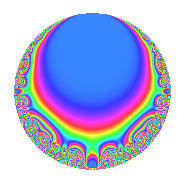# Properties

 Label 7.5.dLevel 7 Weight 5 Character orbit d Rep. character $$\chi_{7}(3,\cdot)$$ Character field $$\Q(\zeta_{6})$$ Dimension 4 Newforms 1 Sturm bound 3 Trace bound 0

# Related objects

## Defining parameters

 Level: $$N$$ = $$7$$ Weight: $$k$$ = $$5$$ Character orbit: $$[\chi]$$ = 7.d (of order $$6$$ and degree $$2$$) Character conductor: $$\operatorname{cond}(\chi)$$ = $$7$$ Character field: $$\Q(\zeta_{6})$$ Newforms: $$1$$ Sturm bound: $$3$$ Trace bound: $$0$$

## Dimensions

The following table gives the dimensions of various subspaces of $$M_{5}(7, [\chi])$$.

Total New Old
Modular forms 8 8 0
Cusp forms 4 4 0
Eisenstein series 4 4 0

## Trace form

 $$4q$$ $$\mathstrut -\mathstrut 4q^{2}$$ $$\mathstrut +\mathstrut 6q^{3}$$ $$\mathstrut -\mathstrut 20q^{4}$$ $$\mathstrut -\mathstrut 30q^{5}$$ $$\mathstrut +\mathstrut 304q^{8}$$ $$\mathstrut -\mathstrut 24q^{9}$$ $$\mathstrut +\mathstrut O(q^{10})$$ $$4q$$ $$\mathstrut -\mathstrut 4q^{2}$$ $$\mathstrut +\mathstrut 6q^{3}$$ $$\mathstrut -\mathstrut 20q^{4}$$ $$\mathstrut -\mathstrut 30q^{5}$$ $$\mathstrut +\mathstrut 304q^{8}$$ $$\mathstrut -\mathstrut 24q^{9}$$ $$\mathstrut -\mathstrut 204q^{10}$$ $$\mathstrut -\mathstrut 58q^{11}$$ $$\mathstrut -\mathstrut 588q^{12}$$ $$\mathstrut +\mathstrut 560q^{14}$$ $$\mathstrut +\mathstrut 468q^{15}$$ $$\mathstrut -\mathstrut 72q^{16}$$ $$\mathstrut -\mathstrut 246q^{17}$$ $$\mathstrut +\mathstrut 216q^{18}$$ $$\mathstrut +\mathstrut 642q^{19}$$ $$\mathstrut -\mathstrut 1050q^{21}$$ $$\mathstrut -\mathstrut 1264q^{22}$$ $$\mathstrut +\mathstrut 290q^{23}$$ $$\mathstrut +\mathstrut 720q^{24}$$ $$\mathstrut -\mathstrut 572q^{25}$$ $$\mathstrut +\mathstrut 1008q^{26}$$ $$\mathstrut -\mathstrut 28q^{28}$$ $$\mathstrut -\mathstrut 2176q^{29}$$ $$\mathstrut -\mathstrut 72q^{30}$$ $$\mathstrut +\mathstrut 3618q^{31}$$ $$\mathstrut +\mathstrut 1584q^{32}$$ $$\mathstrut +\mathstrut 2070q^{33}$$ $$\mathstrut -\mathstrut 2478q^{35}$$ $$\mathstrut -\mathstrut 1632q^{36}$$ $$\mathstrut -\mathstrut 270q^{37}$$ $$\mathstrut -\mathstrut 6168q^{38}$$ $$\mathstrut -\mathstrut 1428q^{39}$$ $$\mathstrut -\mathstrut 1752q^{40}$$ $$\mathstrut +\mathstrut 6048q^{42}$$ $$\mathstrut +\mathstrut 2472q^{43}$$ $$\mathstrut +\mathstrut 2412q^{44}$$ $$\mathstrut +\mathstrut 1944q^{45}$$ $$\mathstrut +\mathstrut 2384q^{46}$$ $$\mathstrut -\mathstrut 1542q^{47}$$ $$\mathstrut -\mathstrut 980q^{49}$$ $$\mathstrut +\mathstrut 7568q^{50}$$ $$\mathstrut -\mathstrut 4734q^{51}$$ $$\mathstrut -\mathstrut 3192q^{52}$$ $$\mathstrut -\mathstrut 4510q^{53}$$ $$\mathstrut -\mathstrut 11016q^{54}$$ $$\mathstrut -\mathstrut 1232q^{56}$$ $$\mathstrut +\mathstrut 11052q^{57}$$ $$\mathstrut -\mathstrut 904q^{58}$$ $$\mathstrut +\mathstrut 2526q^{59}$$ $$\mathstrut -\mathstrut 756q^{60}$$ $$\mathstrut -\mathstrut 282q^{61}$$ $$\mathstrut -\mathstrut 336q^{63}$$ $$\mathstrut -\mathstrut 5472q^{64}$$ $$\mathstrut +\mathstrut 5796q^{65}$$ $$\mathstrut -\mathstrut 2556q^{66}$$ $$\mathstrut -\mathstrut 1318q^{67}$$ $$\mathstrut +\mathstrut 20412q^{68}$$ $$\mathstrut +\mathstrut 3360q^{70}$$ $$\mathstrut -\mathstrut 10408q^{71}$$ $$\mathstrut -\mathstrut 1296q^{72}$$ $$\mathstrut +\mathstrut 5214q^{73}$$ $$\mathstrut +\mathstrut 4036q^{74}$$ $$\mathstrut -\mathstrut 9636q^{75}$$ $$\mathstrut -\mathstrut 8890q^{77}$$ $$\mathstrut -\mathstrut 9072q^{78}$$ $$\mathstrut -\mathstrut 8110q^{79}$$ $$\mathstrut -\mathstrut 3144q^{80}$$ $$\mathstrut +\mathstrut 9306q^{81}$$ $$\mathstrut -\mathstrut 4032q^{82}$$ $$\mathstrut +\mathstrut 588q^{84}$$ $$\mathstrut -\mathstrut 15492q^{85}$$ $$\mathstrut +\mathstrut 12928q^{86}$$ $$\mathstrut +\mathstrut 5976q^{87}$$ $$\mathstrut -\mathstrut 2912q^{88}$$ $$\mathstrut +\mathstrut 33990q^{89}$$ $$\mathstrut +\mathstrut 17640q^{91}$$ $$\mathstrut -\mathstrut 20232q^{92}$$ $$\mathstrut +\mathstrut 7446q^{93}$$ $$\mathstrut +\mathstrut 27768q^{94}$$ $$\mathstrut +\mathstrut 6558q^{95}$$ $$\mathstrut -\mathstrut 37828q^{98}$$ $$\mathstrut +\mathstrut 10368q^{99}$$ $$\mathstrut +\mathstrut O(q^{100})$$

## Decomposition of $$S_{5}^{\mathrm{new}}(7, [\chi])$$ into irreducible Hecke orbits

Label Dim. $$A$$ Field CM Traces $q$-expansion
$$a_2$$ $$a_3$$ $$a_5$$ $$a_7$$
7.5.d.a $$4$$ $$0.724$$ $$\Q(\sqrt{-3}, \sqrt{22})$$ None $$-4$$ $$6$$ $$-30$$ $$0$$ $$q+(-2+\beta _{1}-2\beta _{2})q^{2}+(2-\beta _{1}+\beta _{2}+\cdots)q^{3}+\cdots$$